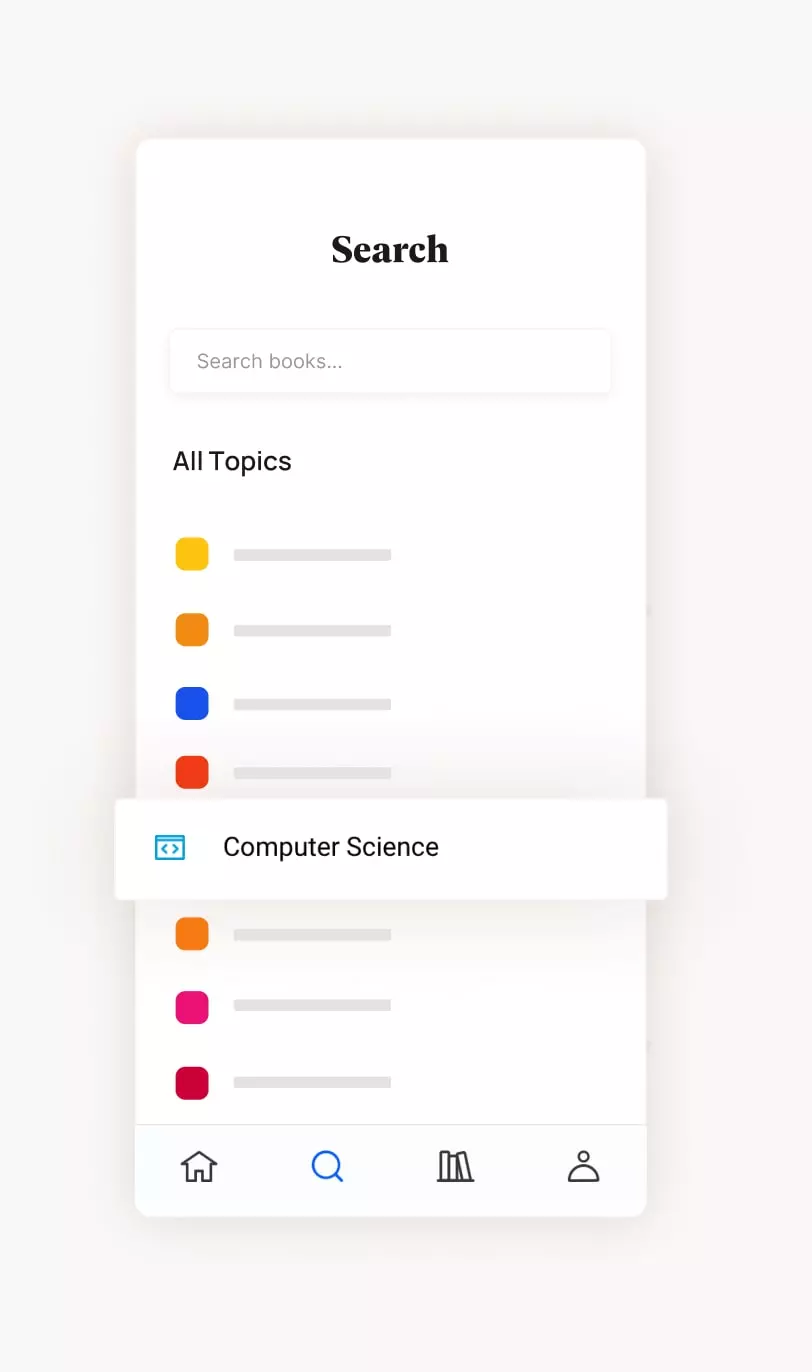[PDF] Partial Differential Equations An Introduction by Walter A. Strauss | Perlego
###### Get access to over 500,000 titles
Start your free trial today and explore our endless library.
Start free trial# Partial Differential Equations

An Introduction
Walter A. Strauss
Start free trialShare book464 pagesEnglishPDFNot available on the Perlego app

# Partial Differential Equations

An Introduction
Walter A. Strauss
Book details
Table of contents

## About This Book

Our understanding of the fundamental processes of the natural world is based to a large extent on partial differential equations (PDEs).  The second edition of Partial Differential Equations provides an introduction to the basic properties of  PDEs and the ideas and techniques that have proven useful in analyzing them.  It provides the student a broad perspective on the subject, illustrates the incredibly rich variety of phenomena encompassed by it, and imparts a working knowledge of the most important techniques of analysis of the solutions of the equations.    In this book mathematical jargon is minimized.  Our focus is on the three most classical PDEs: the wave, heat and Laplace equations.  Advanced concepts are introduced frequently but with the least possible technicalities.  The book is flexibly designed for juniors, seniors or beginning graduate students in science, engineering or mathematics.

Read More

## Information

Publisher
Wiley
Year
2012
ISBN
9781118313169
Topic
Mathematics
Subtopic
Differential Equations
Edition
2